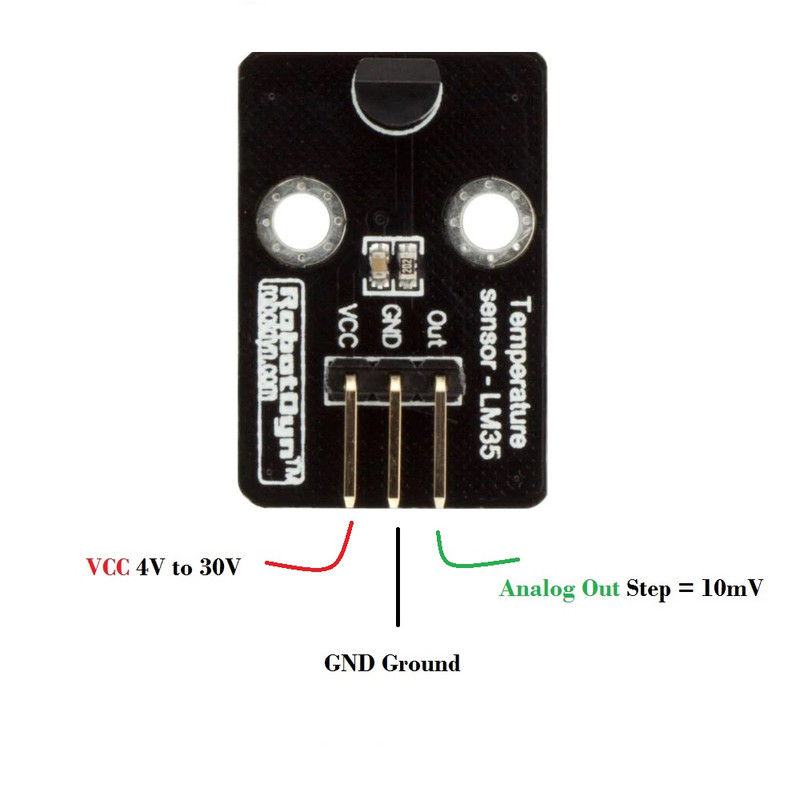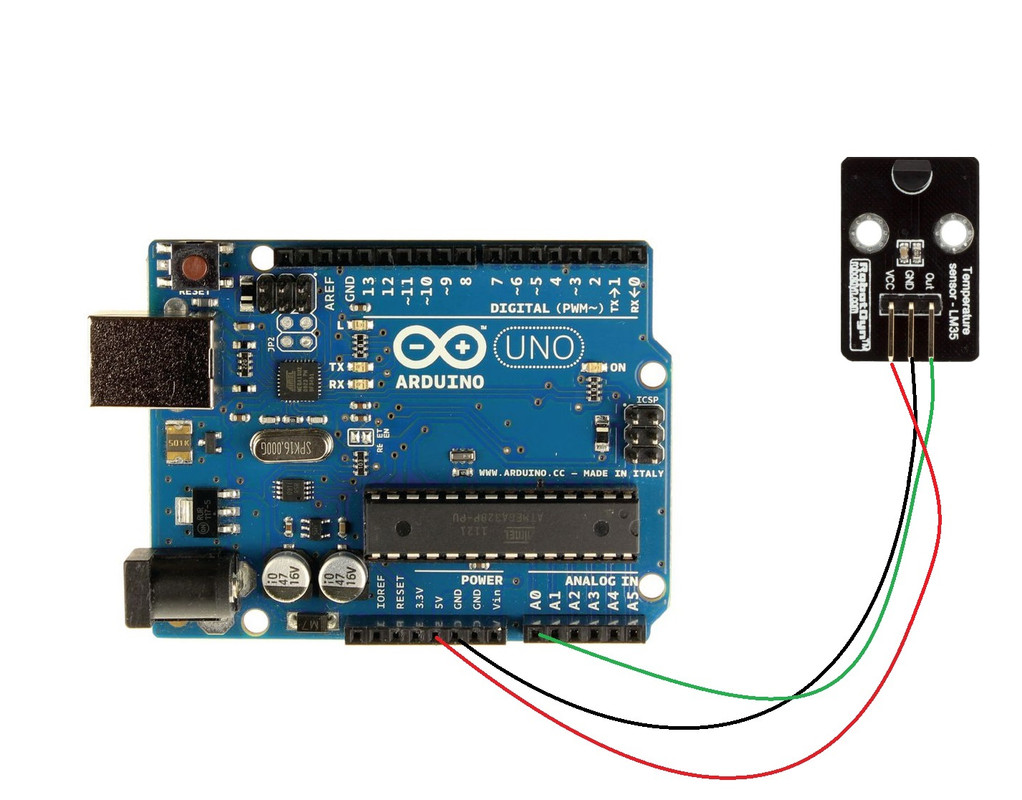## Out Of Stock

1

### Description

The RobotDYN LM35 Temperature Module is an integrated sensor on a board that measures temperature by producing an electrical output signal proportional to the temperature. Accuracy is +/- 0.4 C at room temperature and +/- 0.8 C in the 0 to 100 C range. The LM35 temperature sensor produces a stronger signal than the thermocouple output voltage and does not require output voltage amplification. The current draw is less than 60mA.

Package Includes:

• 1x RobotDYN LM35 Temperature Sensor Module

Features:

• Based on the semiconductor RobotDYN LM35 temperature sensor
• Useful in detecting ambient air temperature
• Calibrated directly in ° Celsius (Centigrade)
• With screw holes for easy installation and fixing. Aperture 2.6mm

Description:

The RobotDYN LM35 is very popular among hobbyists and consumer goods. Texas Instruments introduced the RobotDYN LM35 temperature sensor. It operates by generating an analog signal with reference to the temperature at the resolution of 10mV/0C. It is a compact, low-cost component that is calibrated in Celsius rather than Kelvin, as are other sensors. Its major properties, such as low impedance, linear analog output, and accurate centigrade calibration, make an interface with microcontroller units a simple task.

Principle of Work:

The RobotDYN LM35 measures temperature using solid-state technology. It takes advantage of the voltage drop between the base and emitter of a diode-connected transistor and drops at a predictable pace as temperature rises. It is simple to construct an analog signal that is directly related to temperature by properly magnifying this voltage change. Because of the linear relationship between forward voltage and temperature, diode-connected transistors are utilized as temperature-measuring devices. This is basically how temperature is measured, there have been significant advancements in this technique through the years. All of these sophisticated calculations are performed within the RobotDYN LM35. It simply produces a voltage that is proportional to temperature. The RobotDYN LM35 connecting is easy, just connect the VCC pin to power (4V to 30V) and the GND pin to the ground. Then the Output pin will have an analog voltage that is directly proportional (linear) to the temperature in °C. This can be easily seen in the output voltage vs temperature characteristic. Note that the analog output voltage is independent of the power supply.

To convert the voltage to temperature, simply use the basic formula:

Temperature (°C) = Vout * 100

For example, if the voltage out is 0.5V that means that the temperature is 0.5 * 100 = 50 °C

Pinout of the Board:VCC is the power supply for the sensor which can be anywhere between 4V to 30V.

Analog Out or Vout pin produces an analog voltage that is directly proportional (linear) to the temperature. It should be connected to an Analog (ADC) input.

GND is a ground pin.

Applications:

1. It is used to find the value of temperatures in High volts ac transmission
2. It gives the thermal shutdown for any circuitry
3. It is used for monitoring battery temperature value and saves the battery from the overheating
4. The main application is to find the temperature

Circuit:

we will connect the RobotDYN LM35 Module sensor to the A0 input of the Arduino Uno board and then read the temperature and show it on the serial monitor.Library:

A library is not needed.

Code:

This code is going to print the Temperature sensor on the Serial Monitor.

```#define sensorPin A0

void setup() {
// Begin serial communication at 9600 baud rate
Serial.begin(9600);
}

void loop() {
// Get the voltage reading from the RobotDYN LM35

// Convert that reading into voltage
float voltage = reading * (5.0 / 1024.0);

// Convert the voltage into the temperature in Celsius
float temperatureC = voltage * 100;

// Print the temperature in Celsius
Serial.print("Temperature: ");
Serial.print(temperatureC);
Serial.print("\xC2\xB0"); // shows degree symbol
Serial.print("C  |  ");

// Print the temperature in Fahrenheit
float temperatureF = (temperatureC * 9.0 / 5.0) + 32.0;
Serial.print(temperatureF);
Serial.print("\xC2\xB0"); // shows degree symbol
Serial.println("F");

delay(1000); // wait a second between readings
}
```

Define the Arduino pin that is connected with the out pin of the sensor. After that, in the void setup, initialize the serial monitor by using Serial. begin( ). In the void loop, use the function to read the Analog coming from the sensor. Print the readings by using Serial. Print functions. Also, formulate the programming to return the Celsius readings to Fahrenheit. Give some delay time to get different readings

Technical Details:

• Linear + 10 mV/°C Scale Factor
• 0.5°C Ensure accuracy (at +25°C)
• Low power consumption, less than 60uA
• Low output impedance, 1mA current through only 0.1Ω
• Main chip: RobotDYN LM35 temperature sensor
• Working voltage: DC 4-30V
• The range of temperature measurement: 0---100 C
• The precision of temperature measurement: + 0.5 C
• Wide working voltage range: DC4~30V

Resources:

Tutorial

Comparisons:

The temperature data are measured by the RobotDYN LM35 sensor in Celsius. to evaluate it against the DHT11 sensor While RobotDYN LM35 can only measure temperature, DHT11 can also measure humidity. The RobotDYN LM35 only needs a very little amount of current to operate. The RobotDYN LM35's temperature measurement range, however, is greater than that of the DHT11's to a greater extent. Less voltage is needed for the DHT11 to operate. While the RobotDYN LM35's maximum operating voltage range is 4 to 30 V, it can operate between 3 and 5 V.